# XOR: Neural Networks using TensorFlow¶

## Testing if Tensor Flow NN beats Logistic Regression¶

If you have not already, you might want to make sure you know how to:

### Overview¶

XOR is a classic example that cannot be solved using linear techniques. We put this to the test in this example, where we try to solve XOR using logistic regression, and then solve it using TensorFlow Neural Network method. The data we use is the XOR problem (4 rows of data), repeated multiple times.

### Preliminaries¶

We need to make sure that a few relevant packages have been installed in this environment. If you recall, we created the 'tensorflow_env' environment within Anaconda, and this script is started from within that environment (via JupyterLab). We install the relevant packages below.

In :
#!pip install -q seaborn

In :
#pip install -U scikit-learn


Initiate TensorFlow (tf), and load other libraries that we will need later (e.g. matplotlib, pandas, seaborn)

In :
from __future__ import absolute_import, division, print_function, unicode_literals

import pathlib

import matplotlib.pyplot as plt
import pandas as pd
import seaborn as sns
import numpy as np

try:
# %tensorflow_version only exists in Colab.
%tensorflow_version 2.x
except Exception:
pass
import tensorflow as tf

from tensorflow import keras
from tensorflow.keras import layers

print(tf.__version__)

1.14.0


### Data exploration¶

First we import some sample data. In this case I have taken the typical XOR example, and repeated it 10 times it in a csv file (there are 4 patterns, so 40 total rows). Why 10? Just an arbitrary number to get multiple full-patterns to run NN and LR. Note that I will be using a split model development & model testing sample, and selecting randomly (this is why can't just work with the 4 rows in the XOR example).

Remember in order to execute a 'cell' like the one below, you can 1) click on it and run it using the run button above or 2) click in the cell and hit shift+enter.

In :
import pandas as pd
print(wsmpl.shape) #get (numer of rows, number of columns or 'features')

(40, 3)

In :
#Choose columns to keep (numeric only)
wsmpl_n = wsmpl[['XOR', 'Inp1',	'Inp2']] #XOR is the dependent variable

Out:
XOR Inp1 Inp2
0 0 0 0
1 1 1 0
2 1 0 1
3 0 1 1
4 0 0 0
In :
wsmpl_n.describe() #get some basic stats on the dataset

Out:
XOR Inp1 Inp2
count 40.00000 40.00000 40.00000
mean 0.50000 0.50000 0.50000
std 0.50637 0.50637 0.50637
min 0.00000 0.00000 0.00000
25% 0.00000 0.00000 0.00000
50% 0.50000 0.50000 0.50000
75% 1.00000 1.00000 1.00000
max 1.00000 1.00000 1.00000

See if there is missing data:

In :
wsmpl_n.isna().sum()

Out:
XOR     0
Inp1    0
Inp2    0
dtype: int64

There is no missing data. Good! Let's proceed to split the data into a random 70% for training, and remainder for testing. Remember we did a similar split for the linear regression example using the boston house price dataset.

In :
x_train = wsmpl_n.sample(frac=0.7,random_state=0)
x_test = wsmpl_n.drop(x_train.index)
#specify labels for train/test datasets
y_train  = x_train.pop('XOR')
y_test  = x_test.pop('XOR')


## Logistic Regression¶

We apply logistic regression to the XOR dataset and get performance metrics:

In :
from sklearn.linear_model import LogisticRegression
clf = LogisticRegression(random_state=0, solver='lbfgs', multi_class='multinomial').fit(x_train, y_train)

In :
clf.score(x_train, y_train)

Out:
0.5357142857142857
In :
clf.score(x_test, y_test)

Out:
0.4166666666666667
In :
from sklearn.metrics import confusion_matrix
y_train_true = pd.DataFrame(y_train)
y_train_pred = pd.DataFrame(clf.predict(x_train))
#do the same thing with test dataset
y_test_true = pd.DataFrame(y_test)
y_test_pred = pd.DataFrame(clf.predict(x_test))
pd.DataFrame(confusion_matrix(y_train_true, y_train_pred))

Out:
0 1
0 0 13
1 0 15

That is a pretty bad result! On the training data no-less. No point going to testing data, logistic regression simply can't solve the XOR example. However, for completeness - let's measure on testing data as well, below. Essentially logistic regression classifies every example in the '1' category.

In :
pd.DataFrame(confusion_matrix(y_test_true, y_test_pred))

Out:
0 1
0 0 7
1 0 5

## TensorFlow / NeuralNetworks¶

Let's look at some statistics on the train dataset.

In :
train_stats = x_train.describe()
train_stats = train_stats.transpose()
train_stats

Out:
count mean std min 25% 50% 75% max
Inp1 28.0 0.500000 0.509175 0.0 0.0 0.5 1.0 1.0
Inp2 28.0 0.464286 0.507875 0.0 0.0 0.0 1.0 1.0
In :
train_stats['std'] = np.where(train_stats['std']==0,1,train_stats['std'])
train_stats

Out:
count mean std min 25% 50% 75% max
Inp1 28.0 0.500000 0.509175 0.0 0.0 0.5 1.0 1.0
Inp2 28.0 0.464286 0.507875 0.0 0.0 0.0 1.0 1.0

### Normalize the data¶

Look again at the train_stats block above and note how different the ranges of each feature are.

It is good practice to normalize features that use different scales and ranges. Although the model might converge without feature normalization, it makes training more difficult, and it makes the resulting model dependent on the choice of units used in the input.

Note: Although we intentionally generate these statistics from only the training dataset, these statistics will also be used to normalize the test dataset. We need to do that to project the test dataset into the same distribution that the model has been trained on.

In :
def norm(x):
return (x - train_stats['mean']) / train_stats['std']
normed_train_data = norm(x_train)
normed_test_data = norm(x_test)


This normalized data is what we will use to train the model.

Caution: The statistics used to normalize the inputs here (mean and standard deviation) need to be applied to any other data that is fed to the model, along with the one-hot encoding that we did earlier. That includes the test set as well as live data when the model is used in production.

In :
normed_test_data.head()

Out:
Inp1 Inp2
0 -0.981981 -0.914174
3 0.981981 1.054816
6 -0.981981 1.054816
9 0.981981 -0.914174
12 -0.981981 -0.914174

### The Neural Network Model¶

#### > Build the model¶

Let's build our model. Here, we'll use a Sequential model with two densely connected hidden layers, and an output layer that returns a single, continuous value. The model building steps are wrapped in a function, build_model, since we'll create a second model, later on.

In :
def build_model():
model = keras.Sequential([
layers.Dense(64, activation='relu', input_shape=[len(x_train.keys())]),
layers.Dense(64, activation='relu'),
layers.Dense(1)
])

optimizer = tf.keras.optimizers.RMSprop(0.001)

model.compile(loss='mse',
optimizer=optimizer,
metrics=['mae', 'mse'])
return model

In :
model = build_model();

WARNING:tensorflow:From D:\Data\PredictiveModeler\Anaconda\envs\tensorflow_env\lib\site-packages\tensorflow\python\ops\init_ops.py:1251: calling VarianceScaling.__init__ (from tensorflow.python.ops.init_ops) with dtype is deprecated and will be removed in a future version.
Instructions for updating:
Call initializer instance with the dtype argument instead of passing it to the constructor


#### Inspect the model¶

Use the .summary method to print a simple description of the model

In :
model.summary()

Model: "sequential"
_________________________________________________________________
Layer (type)                 Output Shape              Param #
=================================================================
dense (Dense)                (None, 64)                192
_________________________________________________________________
dense_1 (Dense)              (None, 64)                4160
_________________________________________________________________
dense_2 (Dense)              (None, 1)                 65
=================================================================
Total params: 4,417
Trainable params: 4,417
Non-trainable params: 0
_________________________________________________________________


Now try out the model. Take a batch of 10 examples from the training data and call model.predict on it.

In :
example_batch = normed_train_data[:10]
example_result = model.predict(example_batch)
example_result

Out:
array([[ 0.21917108],
[-0.04631596],
[-0.07782416],
[-0.04631596],
[ 0.21917108],
[ 0.1647872 ],
[-0.04631596],
[ 0.1647872 ],
[ 0.21917108],
[-0.07782416]], dtype=float32)

It seems to be working, and it produces a result of the expected shape and type.

### Train the model¶

Train the model for 1000 epochs, and record the training and validation accuracy in the history object.

Note the validation_split set to use 20% of the training data as validation set and the remainder as calibration. Important to note that this is separate from the testing data that we do not touch in the model-training.

In :
# Display training progress by printing a single dot for each completed epoch
class PrintDot(keras.callbacks.Callback):
def on_epoch_end(self, epoch, logs):
if epoch % 100 == 0: print('')
print('.', end='')

EPOCHS = 1000

history = model.fit(
normed_train_data, y_train,
epochs=EPOCHS, validation_split = 0.2, verbose=0,
callbacks=[PrintDot()])

....................................................................................................
....................................................................................................
....................................................................................................
....................................................................................................
....................................................................................................
....................................................................................................
....................................................................................................
....................................................................................................
....................................................................................................
....................................................................................................

Visualize the model's training progress using the stats stored in the history object.

In :
hist = pd.DataFrame(history.history)
hist['epoch'] = history.epoch
hist.tail()

Out:
loss mean_absolute_error mean_squared_error val_loss val_mean_absolute_error val_mean_squared_error epoch
995 0.000260 0.016028 0.000260 0.000278 0.016579 0.000278 995
996 0.000264 0.016122 0.000264 0.000273 0.016437 0.000273 996
997 0.000261 0.016042 0.000261 0.000286 0.016806 0.000286 997
998 0.000271 0.016310 0.000271 0.000285 0.016785 0.000285 998
999 0.000271 0.016343 0.000271 0.000300 0.017209 0.000300 999
In :
def plot_history(history):
hist = pd.DataFrame(history.history)
hist['epoch'] = history.epoch

plt.figure()
plt.xlabel('Epoch')
plt.ylabel('Mean Abs Error [XOR]')
plt.plot(hist['epoch'], hist['mean_absolute_error'],
label='Train Error')
plt.plot(hist['epoch'], hist['val_mean_absolute_error'],
label = 'Val Error')
plt.ylim([0,5])
plt.legend()

plt.figure()
plt.xlabel('Epoch')
plt.ylabel('Mean Square Error [$XOR^2$]')
plt.plot(hist['epoch'], hist['mean_squared_error'],
label='Train Error')
plt.plot(hist['epoch'], hist['val_mean_squared_error'],
label = 'Val Error')
plt.ylim([0,20])
plt.legend()
plt.show()

plot_history(history)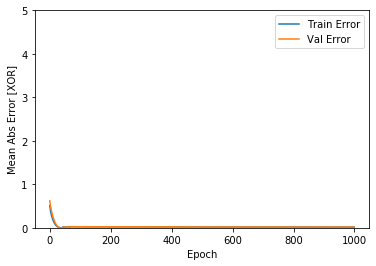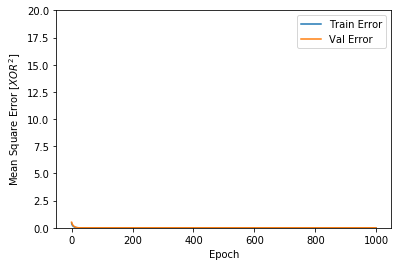This graph shows little improvement in the validation error after about 100 epochs. Let's update the model.fit call to automatically stop training when the validation score doesn't improve further. We'll use an EarlyStopping callback that tests a training condition for every epoch. If a set amount of epochs elapses without showing improvement, then automatically stop the training.

In :
model = build_model()

# The patience parameter is the amount of epochs to check for improvement
early_stop = keras.callbacks.EarlyStopping(monitor='val_loss', patience=10)

history = model.fit(normed_train_data, y_train, epochs=EPOCHS,
validation_split = 0.2, verbose=0, callbacks=[early_stop, PrintDot()])

...............................................

Let's re-plot the history to hopefully see the model training stopping before things get worse for the validation data.

In :
plot_history(history)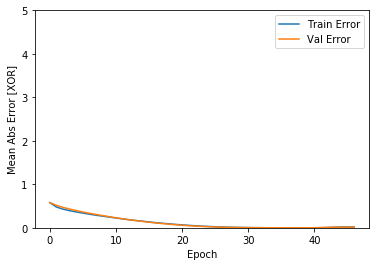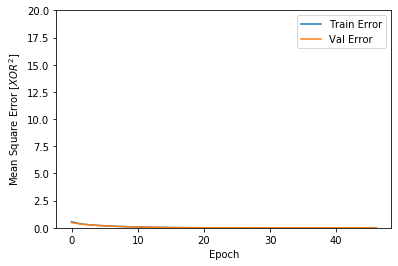The graph shows that on the validation set, the average error is pretty minimal.

Let's see how well the model generalizes by using the test set, which we did not use at all when training the model. This tells us how well we can expect the model to predict when we use it in the real world.

In :
loss, mae, mse = model.evaluate(normed_test_data, y_test, verbose=2)
print("Testing set Mean Abs Error: {:5.2f} XOR".format(mae))

12/12 - 0s - loss: 4.2316e-04 - mean_absolute_error: 0.0188 - mean_squared_error: 4.2316e-04
Testing set Mean Abs Error:  0.02 XOR


### Make predictions¶

Finally, we predict XOR values using data in the testing set (and also training, which we will use in the next step to compute more error metrics):

In :
ypred_test = model.predict(normed_test_data)
ypred_train = model.predict(normed_train_data)

plt.scatter(y_test, ypred_test)
plt.xlabel('True Values [XOR]')
plt.ylabel('Predictions [XOR]')
plt.axis('equal')
plt.axis('square')
plt.xlim([0,plt.xlim()])
plt.ylim([0,plt.ylim()])
_ = plt.plot([-100, 100], [-100, 100])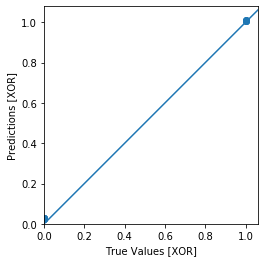In :
from sklearn import metrics
count=0
while (count<7):
count = count + 1
#Training data
ypred_train = pd.DataFrame(model.predict(normed_train_data), columns=['y_pred'])
ypred_train['y_pred'][ypred_train['y_pred'] >= count/10] = 1
ypred_train['y_pred'][ypred_train['y_pred'] <  count/10] = 0
#Test data
ypred_test = pd.DataFrame(model.predict(normed_test_data), columns=['y_pred'])
ypred_test['y_pred'][ypred_test['y_pred'] >= count/10] = 1
ypred_test['y_pred'][ypred_test['y_pred'] <  count/10] = 0
tn, fp, fn, tp = metrics.confusion_matrix(y_test, ypred_test).ravel()
#pd.DataFrame(metrics.confusion_matrix(train_labels, train_pred))
print(f'Count: {count}, train score: {metrics.accuracy_score(y_train, ypred_train)}, \
test score: {metrics.accuracy_score(y_test, ypred_test)}, \
ratio = {tp/(tp+fp)}')

Count: 1, train score: 1.0,     test score: 1.0,     ratio = 1.0
Count: 2, train score: 1.0,     test score: 1.0,     ratio = 1.0
Count: 3, train score: 1.0,     test score: 1.0,     ratio = 1.0
Count: 4, train score: 1.0,     test score: 1.0,     ratio = 1.0
Count: 5, train score: 1.0,     test score: 1.0,     ratio = 1.0
Count: 6, train score: 1.0,     test score: 1.0,     ratio = 1.0
Count: 7, train score: 1.0,     test score: 1.0,     ratio = 1.0

In :
pd.DataFrame(metrics.confusion_matrix(y_train, ypred_train))

Out:
0 1
0 13 0
1 0 15
In :
pd.DataFrame(metrics.confusion_matrix(y_test, ypred_test))

Out:
0 1
0 7 0
1 0 5
In :
#In this code, we denorm testing examples (X), attach the true Y, and then our predictions, and then output that data
data1=x_test
data1['XOR']=y_test
data1['XOR_Pred']=model.predict(normed_test_data)
data1

Out:
Inp1 Inp2 XOR XOR_Pred
0 0 0 0 0.023975
3 1 1 0 0.027154
6 0 1 1 1.008689
9 1 0 1 1.009428
12 0 0 0 0.023975
19 1 1 0 0.027154
21 1 0 1 1.009428
23 1 1 0 0.027154
24 0 0 0 0.023975
26 0 1 1 1.008689
31 1 1 0 0.027154
38 0 1 1 1.008689

### Error analysis¶

The graph above looks pretty, pretty, pretty, good! (pardon the Curb reference!). To get more than a visual understanding of the error, let's compute some error metrics.

We start with developing an empirical distribution of the error term (this is a very useful piece of code!).

In :
error = data1['XOR'] - data1['XOR_Pred']
plt.hist(error, bins = 25)
plt.xlabel("Prediction Error XOR")
_ = plt.ylabel("Count")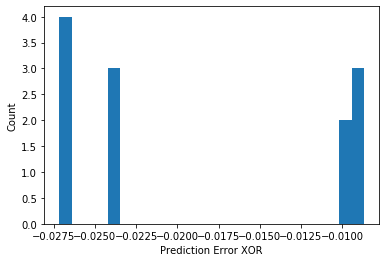Just like we did in the OLS example, let's calculate the mean-squared error, mean absolute error, and the r-squared error on training and testing. This is useful as we can see the extent to which performance degrades from training to testing data (note: we expect this degradation).

In :
from sklearn.metrics import mean_squared_error, mean_absolute_error, r2_score
mse = mean_squared_error(y_test, ypred_test)
print('Mean Squared Error: ',mse)
mae = mean_absolute_error(y_test, ypred_test)
print('Mean Absolute Error: ',mae)
rsq = r2_score(y_train, ypred_train) #R-Squared on the training data
print('R-square, Training: ',rsq)
rsq = r2_score(y_test, ypred_test) #R-Squared on the testing data
print('R-square, Testing: ',rsq)

Mean Squared Error:  0.0
Mean Absolute Error:  0.0
R-square, Training:  1.0
R-square, Testing:  1.0


### Feedback¶

If you have ideas on how to improve this post, please let me know: https://predictivemodeler.com/feedback/